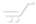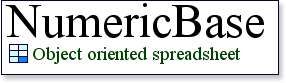# The base rules

The base rules.
The base rules let you to use a single formula to control more than one cell. The chief base rule is called the base row, which is presented in base row page. The two other base rules are the "base column" and the base table.

The base column
The base column is analogue to the base row. A formula located at this column, controls an entire row. To add a base column to your table, add a new column at give it a name that ends with ~base. See the following screenshot for an example: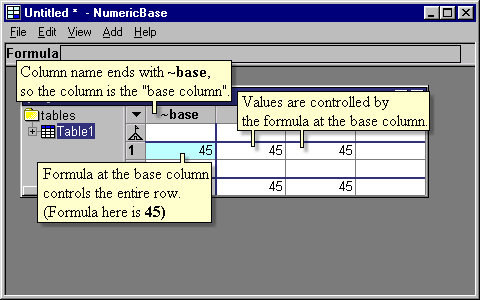Screenshot: the base column.

Range formulas at the base column
Range formulas work in the base column, in the same way as they work in the base row. For example, entering a strong range formula at the base column will generate temporary columns: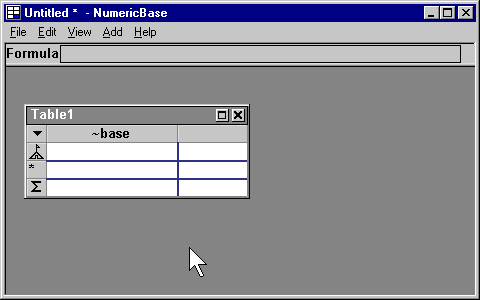Animated screenshot: creating temporary columns

Examples
We have two examples for usage of the base column features: one is a multiplication table, and the other is balance sheet.

Base table
You can define a table that is based on another table. In this context, the first table is called the "derived table" and the second is called the "base table". To define a derived table, use the project window to set its base name attribute to the name of the base table.

For example, in the following screenshot Table2 is based on Table1: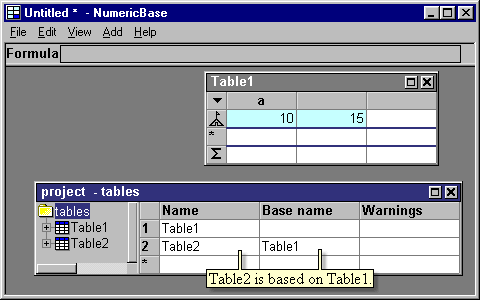Screenshot: base table.

A derived table contains all the columns and rows of the base table. It also let you add any additional columns and rows. The cells of the derived table are controlled by the formulas of corresponding cells at the base table. You can override this behavior for a specific cell by setting the cell formula.Screenshot: Cell uses formula from base table.

The inherited column indicator
Some columns of the derived table are due to columns from the base table. These columns are marked using the inherited column indicator, shown in the following screenshot:Screenshot: the inherited column indicator.

Base rules priorities
In some cases, more than one base rule is applicable for a cell. For example, a cell can be controlled by a formula at the base table, and by a formula at the base row. In this cases, the formula controlling the cell is determined according to the following priorities:
1. The formula of the cell
2. The formula at the same column and the base row.
3. The formula a the same row and the base column.
4. The same formula that control the corresponding cell at the base table. This priority is a recursive definition, because the formula controlling the base table is determined by the same rules.